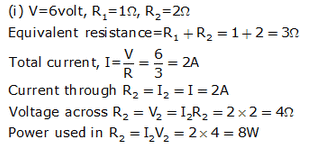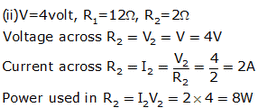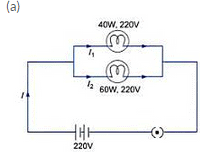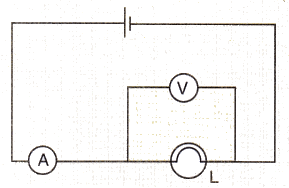Courses

# Solutions of Electricity (Page No- 59) - Physics By Lakhmir Singh, Class 10 Class 10 Notes | EduRev

## Class 10 : Solutions of Electricity (Page No- 59) - Physics By Lakhmir Singh, Class 10 Class 10 Notes | EduRev

The document Solutions of Electricity (Page No- 59) - Physics By Lakhmir Singh, Class 10 Class 10 Notes | EduRev is a part of the Class 10 Course Class 10 Physics Solutions By Lakhmir Singh & Manjit Kaur.
All you need of Class 10 at this link: Class 10

Lakhmir Singh Physics Class 10 Solutions Page No:59

Question 18:
In a house two 60 W electric bulbs are lighted for 4 hours, and three 100 W bulbs for 5 hours everyday. Calculate the electric energy consumed in 30 days.

Solution :

Case 1:
Power, P1 = 60W
Number, t1 = 2
Time for use, t1 = 4h everyday
Electrical energy consumed everyday, E1 = n1 x P1 x t1 = 2 x 60 x 4 = 480 Wh = 0.48kWh
Electrical energy consumed in 30 days = 30 x 0.48 = 14.4kWh

Case 2:
Power, P2 = 100W
Number, n2 = 3
Time for use, t2 = 5h everyday
Electrical energy consumed everyday, E2 = n2 x P2 x t2
= 3x 100 x 5 = 1500 = 1.5kWh
Electrical energy consumed in 30 days = 30x 1.5.45kWh
Total electrical energy consumed in 30 days = 14.4kWh + 45kWh = 59.4kWh

Question 19:
A bulb is rated as 250 V; 0.4 A. Find its : (i) power, and (ii) resistance.

Solution :

V = 250VJ = 0Aarnp
(i) We k now that
Power = VI =250X0.4 = 100watt
(ii) We have
P = I2R
100 = 0.42XR
R = 625ohm

Question 20:
For a heater rated at 4 kW and 220 V, calculate :
(a) the current,
(b) the resistance of the heater,
(c) the energy consumed in 2 hours, and
(d) the cost if 1 kWh is priced at ₹ 60.
Solution :

Given

P = 4kw. V = 220v

(a) 1=?

Power = VI = 250X1

4000 = 2501

I = 16amp

(b) R = ?

p = 12R

P=162XR

R = 4000/162

R = 15.25ohm

(c) Energy consumed in two hour=PXt

= 4X2

= 8kw-hr

(d) If lkwh = Rs 4.6

total cost = 8 x 4.6 = Rs 36.8

Question 21:
An electric motor takes 5 amperes current from a 220 volt supply line. Calculate the power of the motor and electrical energy consumed by it in 2

Solution :

I = 5a mp. V = 220yolt. t = 2 h

P = ?. E = ?

P = VXI

= 220X5

= 1100watt

=1.1kW

Energy consumed, E = PXt

= 1.1X2

= 2.2kWh

Question 22:
Which uses more energy : a 250 W TV set in 1 hour or a 1200 W toaster in 10 minutes ?

Solution :
Case 1: TV set
P = 250W = 0.25kWh
t = lh
Energy consumed = PXt = 0.25X1 = 0.25kWh

Case 2: Toaster
P = 1200W = 1.2kW, t = 10min = 10/60 = 1/6h
Energy consumed = PXt = 1.2X(1/6) = 0.2k1Nh

Thus. TV uses more energy.

Question 23:
Calculate the power used in the 2 Ω resistor in each of the following circuits :
(i) a 6 V battery in series with 1 Ω and 2 Ω resistors.
(ii) a 4 V battery in parallel with 12 Ω and 2 Ω resistors.

Solution :Question 24:
Two lamps, one rated 40 W at 220 V and the other 60 W at 220 V, are connected in parallel to the electric supply at 220 V.
(a) Draw a circuit diagram to show the connections.
(b) Calculate the current drawn from the electric supply.
(c) Calculate the total energy consumed by the two lamps together when they operate for one hour.

Solution : Given 2 lamps:P1 = 40W. P2 = 60W
V = 220V(b) Voltage across both the bulbs is same and is equal to 220V.
Current through 40W lamp = I1 = P1/N = 40/220 A
Current through 60W lamp =I2 = P2N = 60/220 A
Total current drawn from the electric supply = 40/220 + 60/220 = 0.45 A

(a) Energy consumed by 40 W lamp in 1 hr. E1 = P1 x t = 40 x 1 = 40Wh
1Wh = 3.6 kJ
E1 = 40 x 3.6 = 144KJ
Energy consumed by 60W lamp in 1 hr. E2 = P2 x t = 60 x 1= 60Wh = 216 kJ
Total energy consumed = 144 + 216 = 360 kJ

Question 25:
An electric kettle connected to the 230 V mains supply draws a current of 10 A. Calculate :
(a) the power of the kettle.
(b) the energy transferred in 1 minute.

Solution :

Given V = 230V, I = 10amp

(a) P = VI

P = 230X10

P = 2300watt = 2300 Hs

(b) Energy consumed in minute = P x t = 23001/s x 60s = 138000J

Question 26:
A 2 kW heater, a 200 W TV and three 100 W lamps are all switched on from 6m. to 10 p.m. What is the total cost at Rs. 5.50 per kWh ?

Solution :

For heater:
P = 2kW, t = 4h
E = Pxt = 2x4 = 8kWh
For TV:
P = 200W = 0.2kW, t = 4h
E = Pxt = 0.2x4 = 0.8kWh
Lamps:
P = 100W = 0.1kW, t = 4h, n = 3
E = nxPxt = 3x0.1x4 = 1.2kWh
Total energy consumed = 8+0.8+1.2 = 10kWh
Cost of 1kWh = Rs. 5.50
Cost of 10kWh = Rs. 5.50 x 10 = Rs. 55

Question 27:
What is the maximum power in kilowatts of the appliance that can be connected safely to a 13 A ; 230 V mains socket ?

Solution :

I = 13amp, V = 230V

Power = VI

=230X13

= 2990W

P = 2.99kW

Question 28:
An electric fan runs from the 230 V mains. The current flowing through it is 0.4 A. At what rate is electrical energy transferred by the fan ?

Solution : Given :- V = 230V. I = 0.4amp

Rate at which electric energy is transferred = Power

Power = Vxl

= 230 x 0.4

= 92 W

= 92 J/s

Question 29:
(a) What is meant by “electric power” ? Write the formula for electric power in terms of potential difference and current.
(b) The diagram below shows a circuit containing a lamp L, a voltmeter and an ammeter. The voltmeter reading is 3 V and the ammeter reading is 0.5 A(i) What is the resistance of the lamp ?
(ii) What is the power of the lamp ?
(c) Define kilowatt-hour. How many joules are there in one kilowatt-hour ?
(d) Calculate the cost of operating a heater of 500 W for 20 hours at the rate of ? Rs 3.90 per unit.

Solution :

(a) The rate at which electrical work is done or the rate at which electrical energy is consumed, is known as electric power.

It is given by

P = VI = watt

(b) Given: V = 3V, I = 0.5amp

(i) R = ?

We know that V = IR

3 = 0.5R

R = 6ohms

(ii) Power of lamp-VI

= 3x05

= 1.5watt

(c) One kilowatt hour is the amount of electrical energy consumed when an electrical appliance having a power rating of 1 kilowatt is used for 1 hour.

1kWh = 3.6x 1061

(d) Given P = 500W = 0.5kW. t = 20hr

We know that

Energy consumed = Pxt = 0.5X20

= 10kwh

Total cost = 10xcost per uint

Cost per unit = Rs. 3.9 per unit

Therefore. total cost = 10x3.9 = Rs 39

Offer running on EduRev: Apply code STAYHOME200 to get INR 200 off on our premium plan EduRev Infinity!

94 docs

,

,

,

,

,

,

,

,

,

,

,

,

,

,

,

,

,

,

,

,

,

,

,

,

;